# sklearn.linear_model.SGDClassifier¶

class sklearn.linear_model.SGDClassifier(loss='hinge', *, penalty='l2', alpha=0.0001, l1_ratio=0.15, fit_intercept=True, max_iter=1000, tol=0.001, shuffle=True, verbose=0, epsilon=0.1, n_jobs=None, random_state=None, learning_rate='optimal', eta0=0.0, power_t=0.5, early_stopping=False, validation_fraction=0.1, n_iter_no_change=5, class_weight=None, warm_start=False, average=False)[source]

Linear classifiers (SVM, logistic regression, etc.) with SGD training.

This estimator implements regularized linear models with stochastic gradient descent (SGD) learning: the gradient of the loss is estimated each sample at a time and the model is updated along the way with a decreasing strength schedule (aka learning rate). SGD allows minibatch (online/out-of-core) learning via the partial_fit method. For best results using the default learning rate schedule, the data should have zero mean and unit variance.

This implementation works with data represented as dense or sparse arrays of floating point values for the features. The model it fits can be controlled with the loss parameter; by default, it fits a linear support vector machine (SVM).

The regularizer is a penalty added to the loss function that shrinks model parameters towards the zero vector using either the squared euclidean norm L2 or the absolute norm L1 or a combination of both (Elastic Net). If the parameter update crosses the 0.0 value because of the regularizer, the update is truncated to 0.0 to allow for learning sparse models and achieve online feature selection.

Read more in the User Guide.

Parameters:
loss{‘hinge’, ‘log_loss’, ‘log’, ‘modified_huber’, ‘squared_hinge’, ‘perceptron’, ‘squared_error’, ‘huber’, ‘epsilon_insensitive’, ‘squared_epsilon_insensitive’}, default=’hinge’

The loss function to be used.

• ‘hinge’ gives a linear SVM.

• ‘log_loss’ gives logistic regression, a probabilistic classifier.

• ‘modified_huber’ is another smooth loss that brings tolerance to

outliers as well as probability estimates.

• ‘squared_hinge’ is like hinge but is quadratically penalized.

• ‘perceptron’ is the linear loss used by the perceptron algorithm.

• The other losses, ‘squared_error’, ‘huber’, ‘epsilon_insensitive’ and ‘squared_epsilon_insensitive’ are designed for regression but can be useful in classification as well; see SGDRegressor for a description.

More details about the losses formulas can be found in the User Guide.

Deprecated since version 1.0: The loss ‘squared_loss’ was deprecated in v1.0 and will be removed in version 1.2. Use loss='squared_error' which is equivalent.

Deprecated since version 1.1: The loss ‘log’ was deprecated in v1.1 and will be removed in version 1.3. Use loss='log_loss' which is equivalent.

penalty{‘l2’, ‘l1’, ‘elasticnet’}, default=’l2’

The penalty (aka regularization term) to be used. Defaults to ‘l2’ which is the standard regularizer for linear SVM models. ‘l1’ and ‘elasticnet’ might bring sparsity to the model (feature selection) not achievable with ‘l2’.

alphafloat, default=0.0001

Constant that multiplies the regularization term. The higher the value, the stronger the regularization. Also used to compute the learning rate when set to learning_rate is set to ‘optimal’. Values must be in the range [0.0, inf).

l1_ratiofloat, default=0.15

The Elastic Net mixing parameter, with 0 <= l1_ratio <= 1. l1_ratio=0 corresponds to L2 penalty, l1_ratio=1 to L1. Only used if penalty is ‘elasticnet’. Values must be in the range [0.0, 1.0].

fit_interceptbool, default=True

Whether the intercept should be estimated or not. If False, the data is assumed to be already centered.

max_iterint, default=1000

The maximum number of passes over the training data (aka epochs). It only impacts the behavior in the fit method, and not the partial_fit method. Values must be in the range [1, inf).

New in version 0.19.

tolfloat, default=1e-3

The stopping criterion. If it is not None, training will stop when (loss > best_loss - tol) for n_iter_no_change consecutive epochs. Convergence is checked against the training loss or the validation loss depending on the early_stopping parameter. Values must be in the range [0.0, inf).

New in version 0.19.

shufflebool, default=True

Whether or not the training data should be shuffled after each epoch.

verboseint, default=0

The verbosity level. Values must be in the range [0, inf).

epsilonfloat, default=0.1

Epsilon in the epsilon-insensitive loss functions; only if loss is ‘huber’, ‘epsilon_insensitive’, or ‘squared_epsilon_insensitive’. For ‘huber’, determines the threshold at which it becomes less important to get the prediction exactly right. For epsilon-insensitive, any differences between the current prediction and the correct label are ignored if they are less than this threshold. Values must be in the range [0.0, inf).

n_jobsint, default=None

The number of CPUs to use to do the OVA (One Versus All, for multi-class problems) computation. None means 1 unless in a joblib.parallel_backend context. -1 means using all processors. See Glossary for more details.

random_stateint, RandomState instance, default=None

Used for shuffling the data, when shuffle is set to True. Pass an int for reproducible output across multiple function calls. See Glossary. Integer values must be in the range [0, 2**32 - 1].

learning_ratestr, default=’optimal’

The learning rate schedule:

• ‘constant’: eta = eta0

• ‘optimal’: eta = 1.0 / (alpha * (t + t0)) where t0 is chosen by a heuristic proposed by Leon Bottou.

• ‘invscaling’: eta = eta0 / pow(t, power_t)

• ‘adaptive’: eta = eta0, as long as the training keeps decreasing. Each time n_iter_no_change consecutive epochs fail to decrease the training loss by tol or fail to increase validation score by tol if early_stopping is True, the current learning rate is divided by 5.

eta0float, default=0.0

The initial learning rate for the ‘constant’, ‘invscaling’ or ‘adaptive’ schedules. The default value is 0.0 as eta0 is not used by the default schedule ‘optimal’. Values must be in the range (0.0, inf).

power_tfloat, default=0.5

The exponent for inverse scaling learning rate [default 0.5]. Values must be in the range (-inf, inf).

early_stoppingbool, default=False

Whether to use early stopping to terminate training when validation score is not improving. If set to True, it will automatically set aside a stratified fraction of training data as validation and terminate training when validation score returned by the score method is not improving by at least tol for n_iter_no_change consecutive epochs.

New in version 0.20: Added ‘early_stopping’ option

validation_fractionfloat, default=0.1

The proportion of training data to set aside as validation set for early stopping. Must be between 0 and 1. Only used if early_stopping is True. Values must be in the range (0.0, 1.0).

New in version 0.20: Added ‘validation_fraction’ option

n_iter_no_changeint, default=5

Number of iterations with no improvement to wait before stopping fitting. Convergence is checked against the training loss or the validation loss depending on the early_stopping parameter. Integer values must be in the range [1, max_iter).

New in version 0.20: Added ‘n_iter_no_change’ option

class_weightdict, {class_label: weight} or “balanced”, default=None

Preset for the class_weight fit parameter.

Weights associated with classes. If not given, all classes are supposed to have weight one.

The “balanced” mode uses the values of y to automatically adjust weights inversely proportional to class frequencies in the input data as n_samples / (n_classes * np.bincount(y)).

warm_startbool, default=False

When set to True, reuse the solution of the previous call to fit as initialization, otherwise, just erase the previous solution. See the Glossary.

Repeatedly calling fit or partial_fit when warm_start is True can result in a different solution than when calling fit a single time because of the way the data is shuffled. If a dynamic learning rate is used, the learning rate is adapted depending on the number of samples already seen. Calling fit resets this counter, while partial_fit will result in increasing the existing counter.

averagebool or int, default=False

When set to True, computes the averaged SGD weights across all updates and stores the result in the coef_ attribute. If set to an int greater than 1, averaging will begin once the total number of samples seen reaches average. So average=10 will begin averaging after seeing 10 samples. Integer values must be in the range [1, n_samples].

Attributes:
coef_ndarray of shape (1, n_features) if n_classes == 2 else (n_classes, n_features)

Weights assigned to the features.

intercept_ndarray of shape (1,) if n_classes == 2 else (n_classes,)

Constants in decision function.

n_iter_int

The actual number of iterations before reaching the stopping criterion. For multiclass fits, it is the maximum over every binary fit.

loss_function_concrete LossFunction
classes_array of shape (n_classes,)
t_int

Number of weight updates performed during training. Same as (n_iter_ * n_samples).

n_features_in_int

Number of features seen during fit.

New in version 0.24.

feature_names_in_ndarray of shape (n_features_in_,)

Names of features seen during fit. Defined only when X has feature names that are all strings.

New in version 1.0.

sklearn.svm.LinearSVC

Linear support vector classification.

LogisticRegression

Logistic regression.

Perceptron

Inherits from SGDClassifier. Perceptron() is equivalent to SGDClassifier(loss="perceptron", eta0=1, learning_rate="constant", penalty=None).

Examples

>>> import numpy as np
>>> from sklearn.linear_model import SGDClassifier
>>> from sklearn.preprocessing import StandardScaler
>>> from sklearn.pipeline import make_pipeline
>>> X = np.array([[-1, -1], [-2, -1], [1, 1], [2, 1]])
>>> Y = np.array([1, 1, 2, 2])
>>> # Always scale the input. The most convenient way is to use a pipeline.
>>> clf = make_pipeline(StandardScaler(),
...                     SGDClassifier(max_iter=1000, tol=1e-3))
>>> clf.fit(X, Y)
Pipeline(steps=[('standardscaler', StandardScaler()),
('sgdclassifier', SGDClassifier())])
>>> print(clf.predict([[-0.8, -1]]))



Methods

 Predict confidence scores for samples. Convert coefficient matrix to dense array format. fit(X, y[, coef_init, intercept_init, ...]) Fit linear model with Stochastic Gradient Descent. get_params([deep]) Get parameters for this estimator. partial_fit(X, y[, classes, sample_weight]) Perform one epoch of stochastic gradient descent on given samples. Predict class labels for samples in X. Log of probability estimates. Probability estimates. score(X, y[, sample_weight]) Return the mean accuracy on the given test data and labels. set_params(**params) Set the parameters of this estimator. Convert coefficient matrix to sparse format.
decision_function(X)[source]

Predict confidence scores for samples.

The confidence score for a sample is proportional to the signed distance of that sample to the hyperplane.

Parameters:
X{array-like, sparse matrix} of shape (n_samples, n_features)

The data matrix for which we want to get the confidence scores.

Returns:
scoresndarray of shape (n_samples,) or (n_samples, n_classes)

Confidence scores per (n_samples, n_classes) combination. In the binary case, confidence score for self.classes_ where >0 means this class would be predicted.

densify()[source]

Convert coefficient matrix to dense array format.

Converts the coef_ member (back) to a numpy.ndarray. This is the default format of coef_ and is required for fitting, so calling this method is only required on models that have previously been sparsified; otherwise, it is a no-op.

Returns:
self

Fitted estimator.

fit(X, y, coef_init=None, intercept_init=None, sample_weight=None)[source]

Fit linear model with Stochastic Gradient Descent.

Parameters:
X{array-like, sparse matrix}, shape (n_samples, n_features)

Training data.

yndarray of shape (n_samples,)

Target values.

coef_initndarray of shape (n_classes, n_features), default=None

The initial coefficients to warm-start the optimization.

intercept_initndarray of shape (n_classes,), default=None

The initial intercept to warm-start the optimization.

sample_weightarray-like, shape (n_samples,), default=None

Weights applied to individual samples. If not provided, uniform weights are assumed. These weights will be multiplied with class_weight (passed through the constructor) if class_weight is specified.

Returns:
selfobject

Returns an instance of self.

get_params(deep=True)[source]

Get parameters for this estimator.

Parameters:
deepbool, default=True

If True, will return the parameters for this estimator and contained subobjects that are estimators.

Returns:
paramsdict

Parameter names mapped to their values.

partial_fit(X, y, classes=None, sample_weight=None)[source]

Perform one epoch of stochastic gradient descent on given samples.

Internally, this method uses max_iter = 1. Therefore, it is not guaranteed that a minimum of the cost function is reached after calling it once. Matters such as objective convergence, early stopping, and learning rate adjustments should be handled by the user.

Parameters:
X{array-like, sparse matrix}, shape (n_samples, n_features)

Subset of the training data.

yndarray of shape (n_samples,)

Subset of the target values.

classesndarray of shape (n_classes,), default=None

Classes across all calls to partial_fit. Can be obtained by via np.unique(y_all), where y_all is the target vector of the entire dataset. This argument is required for the first call to partial_fit and can be omitted in the subsequent calls. Note that y doesn’t need to contain all labels in classes.

sample_weightarray-like, shape (n_samples,), default=None

Weights applied to individual samples. If not provided, uniform weights are assumed.

Returns:
selfobject

Returns an instance of self.

predict(X)[source]

Predict class labels for samples in X.

Parameters:
X{array-like, sparse matrix} of shape (n_samples, n_features)

The data matrix for which we want to get the predictions.

Returns:
y_predndarray of shape (n_samples,)

Vector containing the class labels for each sample.

predict_log_proba(X)[source]

Log of probability estimates.

This method is only available for log loss and modified Huber loss.

When loss=”modified_huber”, probability estimates may be hard zeros and ones, so taking the logarithm is not possible.

See predict_proba for details.

Parameters:
X{array-like, sparse matrix} of shape (n_samples, n_features)

Input data for prediction.

Returns:
Tarray-like, shape (n_samples, n_classes)

Returns the log-probability of the sample for each class in the model, where classes are ordered as they are in self.classes_.

predict_proba(X)[source]

Probability estimates.

This method is only available for log loss and modified Huber loss.

Multiclass probability estimates are derived from binary (one-vs.-rest) estimates by simple normalization, as recommended by Zadrozny and Elkan.

Binary probability estimates for loss=”modified_huber” are given by (clip(decision_function(X), -1, 1) + 1) / 2. For other loss functions it is necessary to perform proper probability calibration by wrapping the classifier with CalibratedClassifierCV instead.

Parameters:
X{array-like, sparse matrix}, shape (n_samples, n_features)

Input data for prediction.

Returns:
ndarray of shape (n_samples, n_classes)

Returns the probability of the sample for each class in the model, where classes are ordered as they are in self.classes_.

References

Zadrozny and Elkan, “Transforming classifier scores into multiclass probability estimates”, SIGKDD’02, https://dl.acm.org/doi/pdf/10.1145/775047.775151

The justification for the formula in the loss=”modified_huber” case is in the appendix B in: http://jmlr.csail.mit.edu/papers/volume2/zhang02c/zhang02c.pdf

score(X, y, sample_weight=None)[source]

Return the mean accuracy on the given test data and labels.

In multi-label classification, this is the subset accuracy which is a harsh metric since you require for each sample that each label set be correctly predicted.

Parameters:
Xarray-like of shape (n_samples, n_features)

Test samples.

yarray-like of shape (n_samples,) or (n_samples, n_outputs)

True labels for X.

sample_weightarray-like of shape (n_samples,), default=None

Sample weights.

Returns:
scorefloat

Mean accuracy of self.predict(X) wrt. y.

set_params(**params)[source]

Set the parameters of this estimator.

The method works on simple estimators as well as on nested objects (such as Pipeline). The latter have parameters of the form <component>__<parameter> so that it’s possible to update each component of a nested object.

Parameters:
**paramsdict

Estimator parameters.

Returns:
selfestimator instance

Estimator instance.

sparsify()[source]

Convert coefficient matrix to sparse format.

Converts the coef_ member to a scipy.sparse matrix, which for L1-regularized models can be much more memory- and storage-efficient than the usual numpy.ndarray representation.

The intercept_ member is not converted.

Returns:
self

Fitted estimator.

Notes

For non-sparse models, i.e. when there are not many zeros in coef_, this may actually increase memory usage, so use this method with care. A rule of thumb is that the number of zero elements, which can be computed with (coef_ == 0).sum(), must be more than 50% for this to provide significant benefits.

After calling this method, further fitting with the partial_fit method (if any) will not work until you call densify.

## Examples using sklearn.linear_model.SGDClassifier¶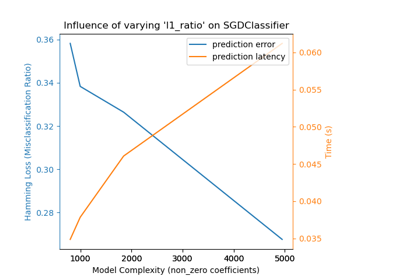Model Complexity Influence

Model Complexity Influence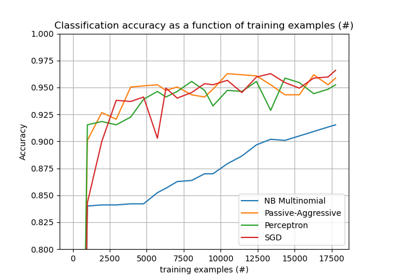Out-of-core classification of text documents

Out-of-core classification of text documents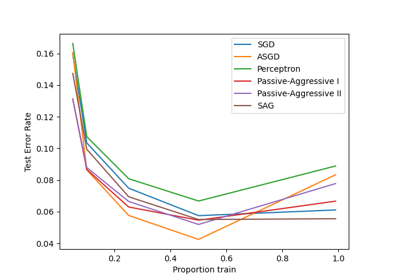Comparing various online solvers

Comparing various online solvers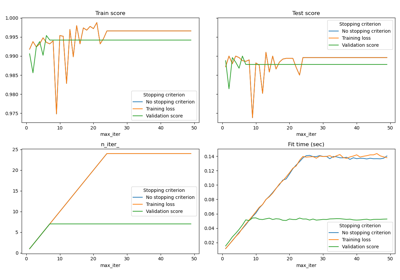Early stopping of Stochastic Gradient Descent

Early stopping of Stochastic Gradient Descent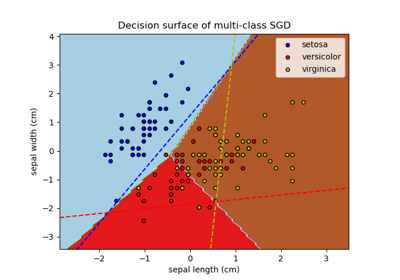Plot multi-class SGD on the iris dataset

Plot multi-class SGD on the iris dataset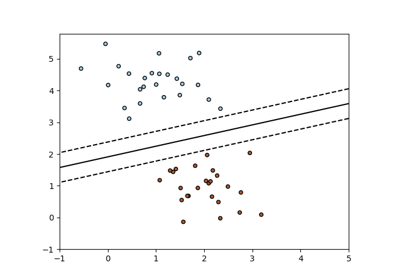SGD: Maximum margin separating hyperplane

SGD: Maximum margin separating hyperplane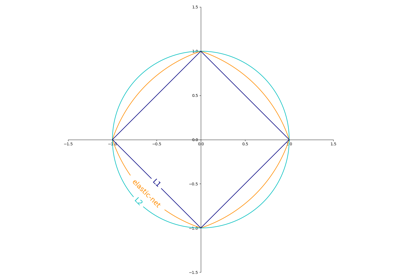SGD: Penalties

SGD: Penalties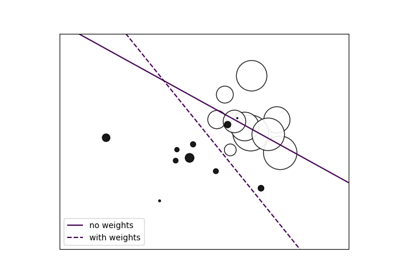SGD: Weighted samples

SGD: Weighted samples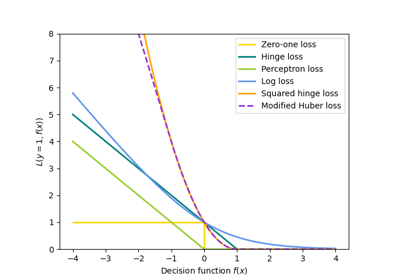SGD: convex loss functions

SGD: convex loss functions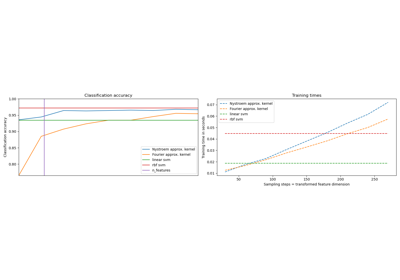Explicit feature map approximation for RBF kernels

Explicit feature map approximation for RBF kernelsComparing randomized search and grid search for hyperparameter estimation

Comparing randomized search and grid search for hyperparameter estimationSample pipeline for text feature extraction and evaluation

Sample pipeline for text feature extraction and evaluationSemi-supervised Classification on a Text Dataset

Semi-supervised Classification on a Text DatasetClassification of text documents using sparse features

Classification of text documents using sparse features